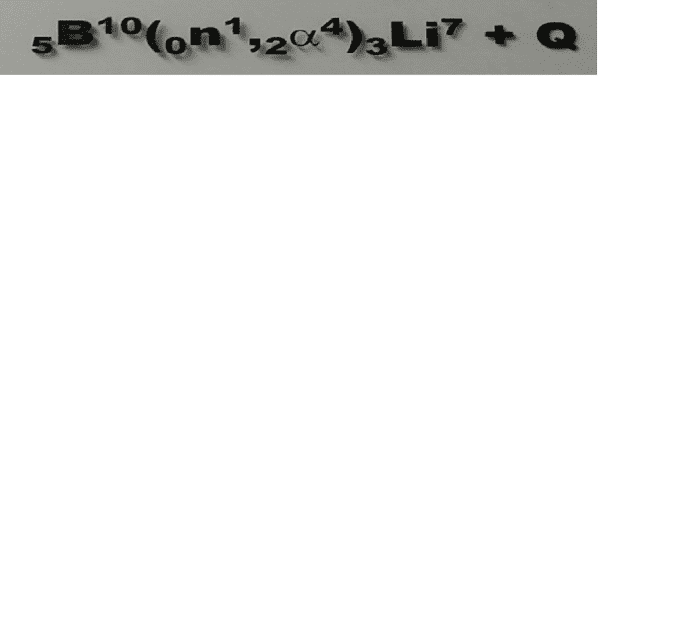# I need help with Nuclear reaction equations

• kokolo

#### kokolo

Homework Statement
I need to prove that number of nucleons is same before and after the reaction.
Relevant Equations
10B(n,α)7Li+ 2.8MeV
En=0 Ek(B)=0 Q=Ek3(α)+Ek4(Li) --Q=Ek3(1+m3/m4) (1)
p3=p4 /^2 -- 2*m3*Ek3=2*m4*Ek4 --- Ek4=m3/m4 * Ek3 (2)
(1) and (2) Ek4= m3/(m3+m4) * Q, but I'm not sure how to find m3 or m4 and if this is true

"I need to prove that number of nucleons is same before and after the reaction" is not a problem statement. It is an expression of your need. Please post the problem statement as was given to you and be sure to include the reaction.

Homework Statement: I need to prove that number of nucleons is same before and after the reaction.
Relevant Equations: 10B(n,α)7Li+ 2.8MeV

En=0 Ek(B)=0 Q=Ek3(α)+Ek4(Li) --Q=Ek3(1+m3/m4) (1)
p3=p4 /^2 -- 2*m3*Ek3=2*m4*Ek4 --- Ek4=m3/m4 * Ek3 (2)
(1) and (2) Ek4= m3/(m3+m4) * Q, but I'm not sure how to find m3 or m4 and if this is true
What are "number of nucleons" in 10B, n, the α-particle, and 7Li?How do you interpret what you posted in #4?
What do you have before the reaction?
What do you have after the reaction?

Also, when you write 5B10, what do the numbers "5" and "10" stand for?